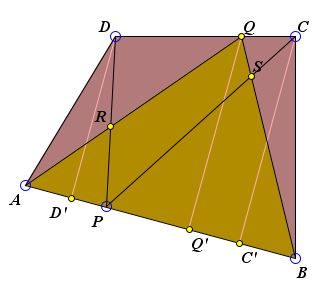# Carpets in a Quadrilateral

Points P and Q are located on the sides AB and CD of a convex quadrilateral ABCD so that AP/AB = CQ/CD. Let S be the intersection of BQ and CP, R the intersection of AQ and DP. Prove that the blue area (quadrilateral PSQR) equals the sum of the red areas (triangles ARD and BSC.)

ExplanationCopyright © 1996-2018 Alexander Bogomolny

This is problem #136 from a problem collection by Barbeau, M. S. Klamkin, W. O. J. Moser. The Carpets Theorem is not mentioned in the book and in fact is not needed in the proof. If anything, the proof supplies an additional demonstration for the most general form of the Carpets Theorem.Denote the common ratio AP/AB = CQ/CD = r. Then

 (1) P = rB + (1 - r)A and Q = rD + (1 - r)C.

Drop perpendiculars CC', QQ' and DD' from C, Q and D to AB and denote their lengths c, q, and d, respectively. Then

q = rd + (1 - r)c.

Now,

Area(ADP) = AP·d / 2 = r·d·AB / 2
Area(BCP) = BP·c / 2 = (1 - r)·c·AB / 2
Area(AQB) = AB·q / 2 = r·d·AB / 2 + (1 - r)·c·AB / 2,

so that obviously

 (2) Area(AQB) = Area(ADP) + Area(BCP).

If we denote AQB as S1 and CPD as T1, we'll find that the most general form of the carpets is directly applicable in our case because of (2) which simply states that

Area(S1) = Area(T2).

The required conclusion follows immediately. However, note that the same result is obtained by subtracting from both sides of (2) the areas of triangles APR and BPS.

### References

1. E. J. Barbeau, M. S. Klamkin, W. O. J. Moser, Five Hundred Mathematical Challenges, MAA, 1995, #136### Carpets TheoremCopyright © 1996-2018 Alexander Bogomolny

68557942